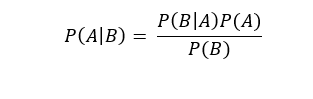# 使用 Scikit-learn 和 ML.NET 实现朴素贝叶斯（Naive Bayes）分类器

Naive Bayes 分类器

Naive Bayes 分类器是一个简单的概率分类器，假定独立变量相互独立，它基于贝叶斯定理，其数学表达如下：ML.NET

var dataPath = "../../../winequality-red.csv";
var ml = new MLContext();
var DataView = ml.Data.LoadFromTextFile<Features>(dataPath, hasHeader: true, separatorChar: ';');

var partitions = ml.Data.TrainTestSplit( DataView,
testFraction: 0.3);
var pipeline = ml.Transforms.Conversion.MapValueToKey(
inputColumnName: "Quality", outputColumnName: "Label")
.Append(ml.Transforms.Concatenate("Features", "FixedAcidity", "VolatileAcidity","CitricAcid", "ResidualSugar", "Chlorides", "FreeSulfurDioxide", "TotalSulfurDioxide","Density", "Ph", "Sulphates", "Alcohol")).AppendCacheCheckpoint(ml);

var trainingPipeline = pipeline.Append(ml.MulticlassClassification.Trainers.NaiveBayes("Label","Features")).Append(ml.Transforms.Conversion.MapKeyToValue("PredictedLabel"));

var trainedModel = trainingPipeline.Fit(partitions.TrainSet);
var testMetrics = ml.MulticlassClassification.Evaluate(trainedModel.Transform(partitions.TestSet));

Scikit-learn

from sklearn.naive_bayes import GaussianNB
from common.import_data import ImportData
from sklearn.model_selection import train_test_split
if __name__ == "__main__":
data_set = ImportData()
x = data_set.import_all_data()
y = data_set.import_columns(np.array(['quality']))

X_train, X_test, y_train, y_test = train_test_split(x, y, test_size=0.2)

NB = GaussianNB()
NB.fit(X_train, y_train.ravel())
predictions = NB.predict(X_test)
print('Scores from each Iteration: ', NB.score(X_test, y_test))

Naive Bayes 分类器用于 Scikit-learn 实现的准确率为 56.5%，而 ML.NET为 41.5%。差异可能是由于其他算法实现方式造成的，但仅基于准确性，我们无法说明哪种方法更好。但是，我们可以说，机器学习算法一种有前途的方式开始出现，即使用 C# 和ML.NET。

posted on 2020-03-26 23:28  Bean.Hsiang  阅读(844)  评论(1编辑  收藏  举报